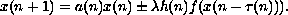Electron. J. Diff. Eqns., Vol. 2002(2002), No. 55, pp. 1-8.

### Positive periodic solutions of nonlinear functional difference equations Youssef N. Raffoul

Abstract:
In this paper, we apply a cone theoretic fixed point theorem to obtain sufficient conditions for the existence of multiple positive periodic solutions to the nonlinear functional difference equationsSubmitted December 22, 2001. Published June 13, 2002.
Math Subject Classifications: 39A10, 39A12.
Key Words: Cone theory, positive, periodic, functional difference equations.

Show me the PDF file (197K), TEX file, and other files for this article.Youssef N. Raffoul Department of Mathematics University of Dayton Dayton, OH 45469-2316 USA e-mail: youssef.raffoul@notes.udayton.edu